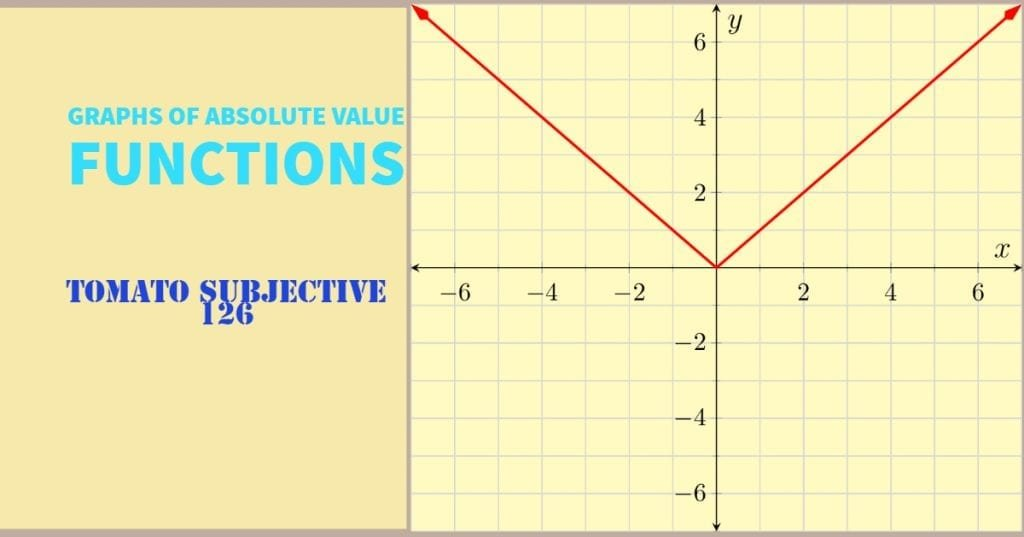How Cheenta works to ensure student success?
Explore the Back-Story

# Test of Mathematics Solution Subjective 126 - Graphs of Absolute Value FunctionsThis is a Test of Mathematics Solution Subjective 126 (from ISI Entrance). The book, Test of Mathematics at 10+2 Level is Published by East West Press. This problem book is indispensable for the preparation of I.S.I. B.Stat and B.Math Entrance.

## Problem

Sketch, on plain paper, the regions represented, on the plane by the following:

(i) $|y| = \sin x$

(ii) $|x| - |y| \ge 1$

## Solution

First we need to understand what |y| signifies. It is the absolute value of y, that is it is +y when y is positive and -y when y is negative.

Lets test with $x = \frac{\pi}{6}$. Clearly then sin x = 1/2. This implies $|y| = 1/2$ or $y = 1/2, -1/2$.

Again let us test with $x = \pi + \frac{\pi}{6}$. Then $\sin (\pi + \frac{\pi}{6}) = - \frac{1}{2}$ implying $|y| = - \frac{1}{2}$. But this is impossible as absolute value cannot be negative.

Using these observations we get a clear idea about what is happening.

• The values of x where sin (x) is positive (that is when $\displaystyle {\frac {4k \pi}{2} \le x \le \frac {(4k+2) \pi }{2} }$, where k is any integer), we draw the graph of y = sin x and reflect it about x axis (as y = sin x and y = - sin x both satisfies the equation).
• The values of x where sin (x) is negative (that is when $\displaystyle {\frac {(4k+2) \pi}{2} \le x \le \frac {(4k+4) \pi }{2} }$, where k is any integer), the given relation is not defined as absolute value of y cannot be negative.For Part (ii)

Notice that |x| gives distance of a point from y axis and |y| gives distance of a point from x axis.

Let us split the problem into cases:

• x, y both non negative (first quadrant). Then |x| = x, |y| = y, implying $|x| - |y| \ge 1$ is same as $x - y \ge 1$• $x \le 0, y \ge 0$ implies |x| = -x. Thus in second quadrant the inequality becomes $-x -y \ge 1$ or $x+y \le -1$• $x \le 0, y \le 0$ implies |x| = -x, |y| = -y. Thus in third quadrant the inequality becomes $-x +y \ge 1$ or $x-y \le -1$• $x \ge 0, y \le 0$ implies |x| = x, |y| = -y. Thus in fourth quadrant the inequality becomes $x + y \ge 1$Hence the final picture is:(only the shaded zones, not the lines).

## Chatuspathi:

• What is this topic: Graphing of functions, inequalities
• What are some of the associated concept: Absolute value functions, inequalities
• Where can learn these topics:  I.S.I. & C.M.I. Entrance Course of Cheenta, discusses these topics in the ‘Calculus’ module.
• Book Suggestions: Play with Graphs (Arihant Publication)This is a Test of Mathematics Solution Subjective 126 (from ISI Entrance). The book, Test of Mathematics at 10+2 Level is Published by East West Press. This problem book is indispensable for the preparation of I.S.I. B.Stat and B.Math Entrance.

## Problem

Sketch, on plain paper, the regions represented, on the plane by the following:

(i) $|y| = \sin x$

(ii) $|x| - |y| \ge 1$

## Solution

First we need to understand what |y| signifies. It is the absolute value of y, that is it is +y when y is positive and -y when y is negative.

Lets test with $x = \frac{\pi}{6}$. Clearly then sin x = 1/2. This implies $|y| = 1/2$ or $y = 1/2, -1/2$.

Again let us test with $x = \pi + \frac{\pi}{6}$. Then $\sin (\pi + \frac{\pi}{6}) = - \frac{1}{2}$ implying $|y| = - \frac{1}{2}$. But this is impossible as absolute value cannot be negative.

Using these observations we get a clear idea about what is happening.

• The values of x where sin (x) is positive (that is when $\displaystyle {\frac {4k \pi}{2} \le x \le \frac {(4k+2) \pi }{2} }$, where k is any integer), we draw the graph of y = sin x and reflect it about x axis (as y = sin x and y = - sin x both satisfies the equation).
• The values of x where sin (x) is negative (that is when $\displaystyle {\frac {(4k+2) \pi}{2} \le x \le \frac {(4k+4) \pi }{2} }$, where k is any integer), the given relation is not defined as absolute value of y cannot be negative.For Part (ii)

Notice that |x| gives distance of a point from y axis and |y| gives distance of a point from x axis.

Let us split the problem into cases:

• x, y both non negative (first quadrant). Then |x| = x, |y| = y, implying $|x| - |y| \ge 1$ is same as $x - y \ge 1$• $x \le 0, y \ge 0$ implies |x| = -x. Thus in second quadrant the inequality becomes $-x -y \ge 1$ or $x+y \le -1$• $x \le 0, y \le 0$ implies |x| = -x, |y| = -y. Thus in third quadrant the inequality becomes $-x +y \ge 1$ or $x-y \le -1$• $x \ge 0, y \le 0$ implies |x| = x, |y| = -y. Thus in fourth quadrant the inequality becomes $x + y \ge 1$Hence the final picture is:(only the shaded zones, not the lines).

## Chatuspathi:

• What is this topic: Graphing of functions, inequalities
• What are some of the associated concept: Absolute value functions, inequalities
• Where can learn these topics:  I.S.I. & C.M.I. Entrance Course of Cheenta, discusses these topics in the ‘Calculus’ module.
• Book Suggestions: Play with Graphs (Arihant Publication)

### Knowledge Partner